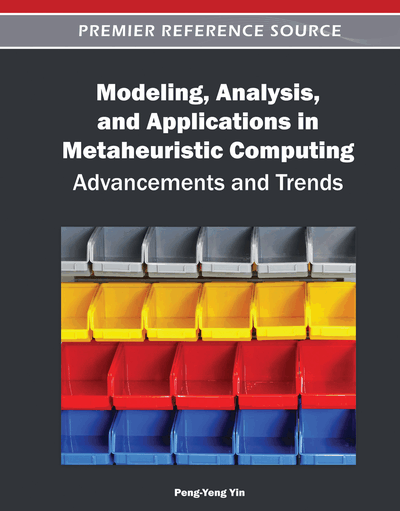# A Reinforcement Learning: Great-Deluge Hyper-Heuristic for Examination Timetabling

Ender Özcan (University of Nottingham, UK), Mustafa Misir (Yeditepe University, Turkey), Gabriela Ochoa (University of Nottingham, UK) and Edmund K. Burke (University of Nottingham, UK)
Copyright: © 2012 |Pages: 22
DOI: 10.4018/978-1-4666-0270-0.ch003
OnDemand PDF Download:
Available
\$37.50
No Current Special Offers

## Abstract

Hyper-heuristics can be identified as methodologies that search the space generated by a finite set of low level heuristics for solving search problems. An iterative hyper-heuristic framework can be thought of as requiring a single candidate solution and multiple perturbation low level heuristics. An initially generated complete solution goes through two successive processes (heuristic selection and move acceptance) until a set of termination criteria is satisfied. A motivating goal of hyper-heuristic research is to create automated techniques that are applicable to a wide range of problems with different characteristics. Some previous studies show that different combinations of heuristic selection and move acceptance as hyper-heuristic components might yield different performances. This study investigates whether learning heuristic selection can improve the performance of a great deluge based hyper-heuristic using an examination timetabling problem as a case study.
Chapter Preview
Top

## 1. Introduction

Meta-heuristics have been widely and successfully applied to many different problems. However, significant development effort is often needed to produce fine tuned techniques for the particular problem or even instance that is under investigation. Hyper-heuristics represent an increasingly popular research direction in search and optimisation (Burke et al., 2003a; Ross, 2005; Chakhlevitch et al., 2008; Özcan et al., 2008; Burke et al. 2009a, 2009b). One of the aims is to at produce more general problem solving techniques, which can potentially be applied to different problems or instances with little development effort. The idea is that a hyper-heuristic approach should able to intelligently choose an appropriate low-level heuristic (from a given repository of heuristics) to be applied at any given time. Thus, in hyper-heuristics, we are interested in adaptively finding solution methods, rather than directly producing a solution for whichever search problem we are studying.

Several hyper-heuristics approaches have been proposed in the literature. It is possible to consider methodologies based on perturbation low-level heuristics and those based on construction low-level heuristics. The latter type builds a solution incrementally, starting with a blank solution and using construction heuristics to gradually build a complete solution. They have been successfully investigated for several combinatorial optimisation problems such as: bin-packing (Tereshima-Marin et al., 2007), timetabling (Terashima-Marin et al., 1999; Burke et al., 2007, Qu et al., 2008), production scheduling (Vazquez-Rodriguez et al., 2007), and cutting stock (Terashima-Marin et al., 2005). On the other hand, approaches based on perturbation heuristics find a reasonable initial solution by some straightforward means (either randomly or using a simple construction heuristic) and then use heuristics, such as shift and swap to perturb solution components with the aim of finding improved solutions. In other words, they start from a complete solution and then search or select among a set of neighbourhoods for better solutions. A class of the most commonly used hyper-heuristics based on perturbation (improvement) low level heuristics is the choice hyper-heuristics (heuristic selection methodologies). They have been applied to real world problems, such as, personnel scheduling (Cowling et al., 2001; Burke et al., 2003b), timetabling (Burke et al., 2003b; Dowsland et al., 2007), and vehicle routing problems (Pisinger et al., 2007). In a choice hyper-heuristic framework based on perturbation low level heuristics, search is mostly performed using a single candidate solution. Such hyper-heuristics, iteratively, attempt to improve a given solution throughout two consecutive phases: heuristic selection and move acceptance as illustrated in Figure 1.

Figure 1.

A hyper-heuristic framework based on a single point search, where St denotes a candidate solution at time t, Hi is the ith low level heuristic, Rt is the resultant solution after applying a set of selected low level heuristics that goes into the move acceptance process

In Figure 1, a candidate solution (St) at a given time (t) is modified into a new solution (or solutions) using a chosen heuristic (or heuristics). Then, a move acceptance method is employed to decide whether to accept or reject a resultant solution (Rt). This process is repeated until a predefined stopping condition is met. Only problem independent information flow is allowed between the problem domain and hyper-heuristic layers. Unless, we specifically say otherwise, a choice hyper-heuristic refers to a hyper-heuristic that operates on a set of perturbation low level heuristics from this point onwards. Moreover, such a hyper-heuristic will be denoted as heuristic selectionmove acceptance based on its components.

## Complete Chapter List

Search this Book:
Reset